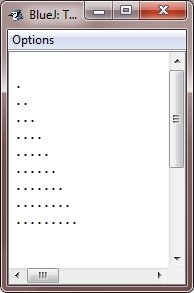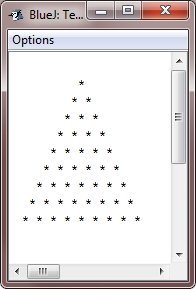# for loop in Java with examples

Iterating efficiently over a set of values is a fundamental programming task, and the for loop is one of the most frequently used Java constructs for this purpose. This article examines the syntax and features of the for loop in Java and demonstrates how it can be used to simplify code and increase productivity.

A "for" loop in Java is a control flow statement that allows you to repeat a block of code a predetermined number of times. It is useful when you know the number of times you wish to execute a block of code or when you wish to traverse a range of values.

Note: The enhanced and nested "for" loops are defined at the bottom of this post.

## Java for loop syntax

The general form, or syntax, of the "for" loop in Java is as follows:

```for (initialization; condition; update) {
// body of the "for" loop
}```

During "initialization," we declare and initialize all variables that will be used in the loop. This is done only once before the loop begins.

The "condition" is an evaluation of a boolean expression performed before each iteration of the loop. If the condition is met, the iteration will continue. If it is false, the loop terminates.

In the final step, "update," any variables declared in the initialization step are updated. This is typically performed at the conclusion of each loop iteration.

As an example:

Java Code
```public class ForLoopExample {
public static void main(String[] args) {
for (int i = 1; i <= 5; i++) {
System.out.println("The value of i is: " + i);
}
}
}```
Output
```The value of i is: 1
The value of i is: 2
The value of i is: 3
The value of i is: 4
The value of i is: 5```

The dry run of the "for" loop used in the above program goes in this way:

• Because the "initialization" executes first and only once. Therefore, an integer variable "i" was declared and initialized with 1. Therefore "i = 1".
• Now, before entering the body of the loop, the condition (i <= 5) must be satisfied. Because at first, the condition (1 <= 5) evaluates to true, the program enters the body of the loop.
• Inside the body of the loop, the System.out.printl() statement is executed, which prints the value of "i" on the output console.
• Since after each iteration of the loop, the "update" statement executes. Therefore, after executing the block of code written inside the loop's body, the "i++" statement executes, which increments the value of "i". Therefore, "i = 2" now.
• Again, before entering the body of the loop, the condition with the new value of "i" gets evaluated. At the second time also, the condition (2 <= 5) evaluates to true. Therefore, program flow again enters the loop's body.
• This process continues until the specified condition evaluates to false.

In other words

• Initialization: int i = 1 initializes a variable i to 1 before the loop starts.
• Condition: i <= 5 specifies the condition that must be true for the loop to continue executing.
• Increment: i++ increments the value of i by 1 at the end of each loop iteration.

## Java for loop example

Consider the following Java program as an illustration of the "for" loop: Because we typically use the "for" loop to print array elements, I'll use the following Java program to demonstrate array element printing using the "for" loop:

Java Code
```public class JavaForLoopExample {
public static void main(String[] args) {
int[] nums = {1, 2, 3, 4, 5, 6, 7, 8, 9, 10};
for (int i = 0; i < nums.length; i++) {
System.out.println(nums[i]);
}
}
}```
Output
```1
2
3
4
5
6
7
8
9
10```

Because indexing in arrays starts at 0, nums " refers to the first element (1), "nums " refers to the second element (2), and so on.

Now, let's change the above program to allow the user to specify the size and elements of the array using the "for" loop, and then, of course, print the element back on the output console using the "for" loop.

Java Code
```import java.util.Scanner;

public class JavaForLoopExample {
public static void main(String[] args) {
Scanner input = new Scanner(System.in);

// ask the user for the size of the array
System.out.print("Enter the size of the array: ");
int size = input.nextInt();

// create an array with the given size
int[] numbers = new int[size];

// ask the user to enter each element of the array
for (int i = 0; i < size; i++) {
System.out.print("Enter element #" + (i+1) + ": ");
numbers[i] = input.nextInt();
}

// print out the elements of the array using a "for" loop
System.out.println("\nThe elements of the array are:");
for (int i = 0; i < size; i++) {
System.out.println(numbers[i]);
}
}
}```
Output
```Enter the size of the array: 4
Enter element #1: 10
Enter element #2: 20
Enter element #3: 30
Enter element #4: 40
Enter element #5: 50

The elements of the array are:
10
20
30
40
50```

## for each or enhanced for loop in Java

A variation of the standard "for" loop made specifically for iterating over arrays and collections is the "for each" or enhanced for loop in Java. It is frequently used in Java programming and offers a streamlined syntax that makes it simpler to iterate over the components of a collection.

The enhanced for loop has the following syntax:

```for (type var : array) {
// loop's body
}```

In this syntax, "type" denotes the type of the array's elements, "var" denotes the variable that will temporarily store each element of the array, and array denotes the array or collection that we want to iterate over. As an example:

Java Code
```public class JavaEnhancedForLoopExample {
public static void main(String[] args) {
int[] nums = {1, 2, 3, 4, 5, 6, 7, 8, 9, 10};

for (int x : nums) {
System.out.println(x);
}
}
}```
Output
```1
2
3
4
5
6
7
8
9
10```

## Java's nested "for" loop

We can nest one "for" loop inside another in the Java programming language. As an example:

```public class JavaProgram {
public static void main(String args[]) {
int i, j;

for (i = 0; i < 10; i++) {
for (j = 0; j < i; j++) {
System.out.print(".");
}
System.out.println();
}
}
}```

This program contains nested "for" loops. The outer loop sets "i" to 0 and continues looping as long as "i" is less than 10. The inner loop is executed on each iteration, which initializes "j" to 0 and continues looping as long as "j" is less than "i." Every time the inner loop iterates, a period (.) is printed to the console.

When the above Java program is compiled and executed, it will produce the following output:Let's look at one more example that also demonstrates the nested loops:

```public class JavaProgram {
public static void main(String args[]) {

int i, j, k, space = 10;

for (i = 0; i < 10; i++) {
for (k = 0; k < space; k++) {
System.out.print(" ");
}

for (j = 0; j < i; j++) {
System.out.print("* ");
}
System.out.println();

space--;
}

}
}```

The sample run of the above Java program is shown here:Java Online Test

« Previous Tutorial Next Tutorial »